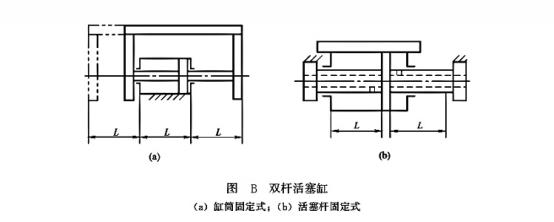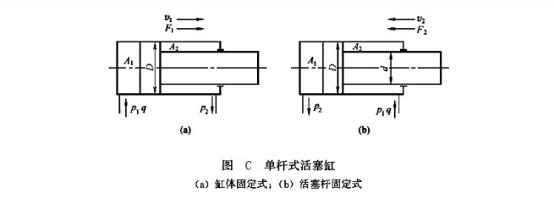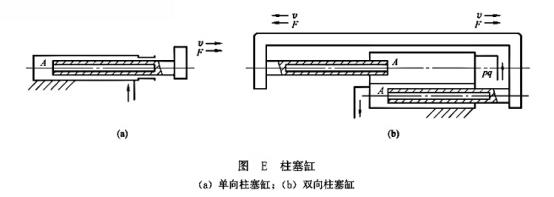Created on：2022-04-09 09:33

# Piston hydraulic cylinder and plunger hydraulic cylinder

Piston hydraulic cylinder and plunger hydraulic cylinder

Piston hydraulic cylinders can be divided into two types: double rod type and single rod type according to their different application requirements.

(1)       Double rod piston cylinder. Double rod piston cylinder is a piston rod with equal diameter extending from both ends of the piston. According to different installation methods, it can be divided into cylinder fixed type and piston rod fixed type. Figure B (a) shows a double rod piston cylinder with fixed cylinder barrel. Its oil inlet and outlet are arranged at both ends of the cylinder barrel. The piston drives the worktable to move through the piston rod. When the effective stroke of the piston is l, the movement range of the whole worktable is 3L. Therefore, the machine tool covers a large area and is generally suitable for small machine tools. When the stroke of the worktable is required to be long, the fixed form of piston rod shown in Figure B (b) can be adopted. The cylinder block is connected with the worktable, the piston rod is fixed on the machine tool through the support, and the power is transmitted from the cylinder block. In this installation form, the moving range of the worktable is only equal to 2 times (2L) of the effective stroke l of the hydraulic cylinder, so the floor area is small. The oil inlet and outlet ports can be set at both ends of the fixed hollow piston rod, but they must be connected with hoses.Since the diameters of the piston rods at both ends of the double rod piston cylinder are usually equal, the effective areas of the left and right cavities are also equal. When the oil with the same pressure and flow rate is input into the left and right chambers respectively, the thrust and speed in the left and right directions of the hydraulic cylinder are equal. The diameter of the piston is D, the diameter of the piston rod is D, the pressure of the oil inlet and outlet chambers of the hydraulic cylinder is P1 and P2, the input flow is Q, and the thrust F and speed port of the double rod piston cylinder are

F1=F2=A(p1-p2)=(π/4)(D2-d2)(p1-p2) (5-1)

v1=v2=q/A=4q/π(D2-d2) (5-2)

Where, D is the diameter of piston rod; D is the piston diameter; P1 and P2 are the working pressure of the oil inlet and outlet chambers of the hydraulic cylinder, and Q is the flow of the input hydraulic cylinder.

When the double rod piston cylinder works, it is designed that one piston rod is under tension and the other piston rod is not under force, so the piston rod of this hydraulic cylinder can be made thinner.

(2) Single rod piston cylinder. As shown in Figure C, the piston has only one end with a piston rod, and the single rod piston cylinder also has two forms of cylinder block fixation and piston rod fixation, but their workbench movement range is twice the effective stroke of the piston.Because the effective working areas of the two chambers of the hydraulic cylinder are different, its output thrust and speed in both directions are also different, and their values are

F1=(p1A1-p2A2)=π/4[p1D2-p2(D2-d2)] (5-3)

v1=q/A1=4q/πD2 (5-4)

F2=p1A2-p2A1=π/4[p1(D2-d2)-p2D2] (5-5)

v2=q/A2=4q/π(D2-d2) (5-6)

Where, D is the diameter of piston rod; D is the piston diameter; P1 is the working pressure of the hydraulic cylinder; Q is the flow of the input hydraulic cylinder.

From formula (5-3) to formula (5-6), since A1 > A2, F1 > F2 and V1 < v2. If the ratio of the output speeds V2 and V1 in both directions is called the speed ratio, it is recorded as φ， be

Φ= v2/v1=[4q/π(D2-d2)]/(4q/πD2) (5-7)

Therefore, the smaller the diameter of the piston rod, Φ The closer it is to 1, the smaller the speed difference between the two directions of the piston. If the piston rod is thick, the speed difference between the two directions of piston movement is large, which can be used for rapid retraction movement. In known D and Φ In this case, D can be easily determined, i.e

D=D√￣[( Φ- 1)/ Φ] （5-8）

(3) Differential cylinder. If the left and right cavities of the single rod piston cylinder are connected with oil pipes and high-pressure oil is introduced at the same time, that is, differential connection, as shown in Figure D. The single rod hydraulic cylinder with differential connection is called differential hydraulic cylinder. When it starts working, the oil pressure in the left and right chambers of the dynamic cylinder is the same. However, since the effective area of the left chamber (without rod chamber) is greater than that of the right chamber (with rod chamber), the piston moves to the right, and the oil discharged from the right chamber (flow Q ') also enters the left chamber, increasing the flow into the left chamber (Q + Q'), This also speeds up the movement of the piston. In fact, when the piston is moving, due to the pressure loss in the pipeline between the two chambers during differential connection, the oil pressure in the right chamber is slightly greater than that in the left chamber, and this difference is generally small and can be ignored. Therefore, the piston thrust F3 and movement speed V3 during differential connection are

F3=p1(A1-A2)=p1(πd2/4) (5-9)

The flow into the rodless cavity is

Q1=v3(πD2/4)=q+v3[π(D2-d2)/4] (5-10)

Where, D is the diameter of piston rod; D is the piston diameter; P1 is the working pressure of the hydraulic cylinder; Q is the pump output flow.

According to formula (5-9) and formula (5-10), the thrust of hydraulic cylinder in differential connection is smaller than that in non differential connection, and the speed is larger than that in non differential connection. Using this, we can get a faster movement speed without increasing the oil source flow. This connection method is widely used in the rapid movement of hydraulic power system of modular machine tools and other mechanical equipment. If the round-trip speed of the machine tool is required to be equal, even if V1 = V2, it can be obtained from equations (5-6) and (5-10)

4q / [π (d2-d2)] = 4q / π D2, i.e. d = (√ ￣ 2) d (5-11)

2. Plunger cylinder

Figure e (a) shows a one-way plunger cylinder, which can only realize hydraulic transmission in one direction, and the reverse movement depends on external force. If bidirectional motion is required, it must be used in pairs, as shown in Figure e (b). The plunger in the hydraulic cylinder is not in contact with the cylinder barrel, and is guided by the guide sleeve on the cylinder head during movement. Therefore, the inner wall of the cylinder barrel does not need finishing, and it will not be suitable for occasions with long stroke.The thrust and speed output by the plunger cylinder are

F=pA=p(πd2/4) (5-12)

V=q/A=4q/πd2 (5-13)

Where, D is the plunger diameter and P is the pressure input to the plunger cylinder; Q is the flow input to the plunger cylinder.

Home    Article    Piston hydraulic cylinder and plunger hydraulic cylinder